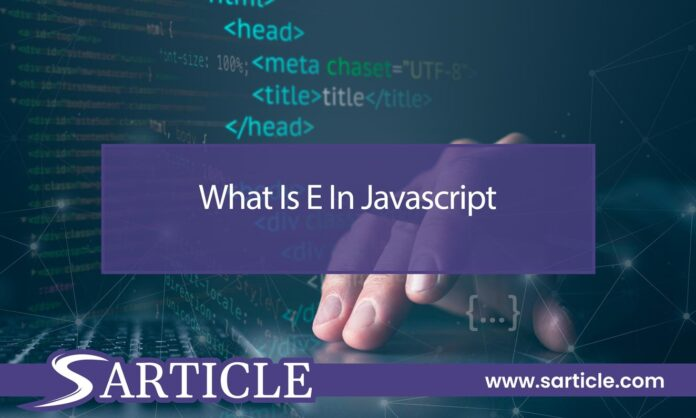# What Is E in Javascript

0
52What Is E in Javascript. In Javascript: scientific notation, handling numbers, precision, and practical uses. Learn about “E” in Javascript today.

Javascript, the versatile programming language used extensively for web development, comes with its own set of peculiarities and notations. One such notation is the letter E which holds significance in numeric representations. In this article, we will delve into the role of “E” in Javascript and its impact on handling large and small numbers.

As developers work with numbers in Javascript, they often encounter situations where expressing extremely large or small values can be challenging. The “E” notation provides an elegant solution for representing such values in a compact and manageable format. Let’s dive into how “E” is utilized and its implications for numerical operations.

## The Notation “E”

In Javascript, “E” represents the exponent in scientific notation. Scientific notation is a concise way of expressing numbers, especially those with many leading or trailing zeros. It consists of two parts: the significand (also known as the mantissa) and the exponent.

## Scientific Notation in Javascript

Scientific notation in Javascript is used to handle numbers that are either too large or too small to be conveniently written in standard decimal notation. The “E” notation separates the significand from the exponent with the letter “E,” indicating the power of 10 by which the significand is multiplied.

## Exponential Representation

For instance, the number 6.022 x 10^23 (Avogadro’s number) can be represented in Javascript as 6.022e23. Similarly, a small number like 0.00000000000000000016 can be expressed as 1.6e-19.

## Dealing with Large and Small Numbers

“E” notation is particularly useful when dealing with astronomical figures, such as distances between celestial bodies, or microscopic measurements in science. It enables developers to work with these numbers without losing precision or encountering issues due to the limitations of standard floating-point representation.

## Floating-Point Arithmetic

Javascript uses floating-point arithmetic to represent numbers, which can sometimes lead to rounding errors or imprecisions. While “E” notation helps manage these scenarios better, it’s important to remain aware of the limitations of floating-point arithmetic and employ appropriate techniques for accurate calculations.

## Precision Considerations

While “E” notation aids in handling large and small numbers, precision remains a consideration. Depending on the magnitude of the exponent, calculations might encounter limitations due to the finite storage capacity of floating-point representations.

## Practical Applications

“E” notation finds applications in various domains, including physics, engineering, finance, and more. Whether calculating distances between galaxies, modeling particle interactions, or dealing with currency values, “E” notation allows programmers to work with diverse data ranges.

## Common Mistakes and Misconceptions

Novice developers might sometimes misinterpret “E” notation as a variable or an error in their code. It’s essential to educate oneself about this notation to prevent misunderstandings and ensure accurate programming.

## Conclusion

Understanding the role of “E” notation in Javascript is crucial for any developer working with numbers, especially those involving vast or minute values. By utilizing “E” notation, programmers can tackle complex calculations without compromising precision and readability, making it an essential tool in the realm of numerical programming.

## Is "E" notation specific to Javascript?

No, “E” notation is a standard notation used in various programming languages and scientific contexts to represent numbers in exponential form.

## Can "E" notation be used with non-numeric values?

No, “E” notation is designed for numeric values and their exponents.

## Are there alternatives to "E" notation for handling large and small numbers?

Yes, some languages offer alternatives like “D” notation, but “E” notation is widely used and understood.

## Can "E" notation result in loss of precision?

While “E” notation helps manage precision, it doesn’t eliminate the challenges associated with finite floating-point representations.